﻿ MakeGameServer - How to find my Internal IP Address. How can I find out my Internal IP Address in my network.
 (function(d, s, id) { var js, fjs = d.getElementsByTagName(s); if (d.getElementById(id)) return; js = d.createElement(s); js.id = id; js.src = "//connect.facebook.net/en_US/all.js#xfbml=1&appId=113303222126557"; fjs.parentNode.insertBefore(js, fjs); }(document, 'script', 'facebook-jssdk')); Tweet

# Make Game Server - How to find out my Internal IP Address

## How to find my Internal IP Address.How can I find out my Internal IP Address in my network.

##### Author: S.P.E.C.1.@.L. - (Max Maxymenko) #Author { color: #00F; } #This { font-weight: bold; color: #F00; } function MM_swapImgRestore() { //v3.0 var i,x,a=document.MM_sr; for(i=0;a&&i<a.length&&(x=a[i])&&x.oSrc;i++) x.src=x.oSrc; } function MM_preloadImages() { //v3.0 var d=document; if(d.images){ if(!d.MM_p) d.MM_p=new Array(); var i,j=d.MM_p.length,a=MM_preloadImages.arguments; for(i=0; i<a.length; i++) if (a[i].indexOf("#")!=0){ d.MM_p[j]=new Image; d.MM_p[j++].src=a[i];}} } function MM_findObj(n, d) { //v4.01 var p,i,x; if(!d) d=document; if((p=n.indexOf("?"))>0&&parent.frames.length) { d=parent.frames[n.substring(p+1)].document; n=n.substring(0,p);} if(!(x=d[n])&&d.all) x=d.all[n]; for (i=0;!x&&i<d.forms.length;i++) x=d.forms[i][n]; for(i=0;!x&&d.layers&&i<d.layers.length;i++) x=MM_findObj(n,d.layers[i].document); if(!x && d.getElementById) x=d.getElementById(n); return x; } function MM_swapImage() { //v3.0 var i,j=0,x,a=MM_swapImage.arguments; document.MM_sr=new Array; for(i=0;i<(a.length-2);i+=3) if ((x=MM_findObj(a[i]))!=null){document.MM_sr[j++]=x; if(!x.oSrc) x.oSrc=x.src; x.src=a[i+2];} } This info is FREE to print, if you want to copy any of  our information, you have to include the link of our Website into your copied text. Author: [email protected]. - (Max Maxymenko)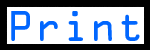How to find out my Internal IP Address

1. Go to START => RUN => CMD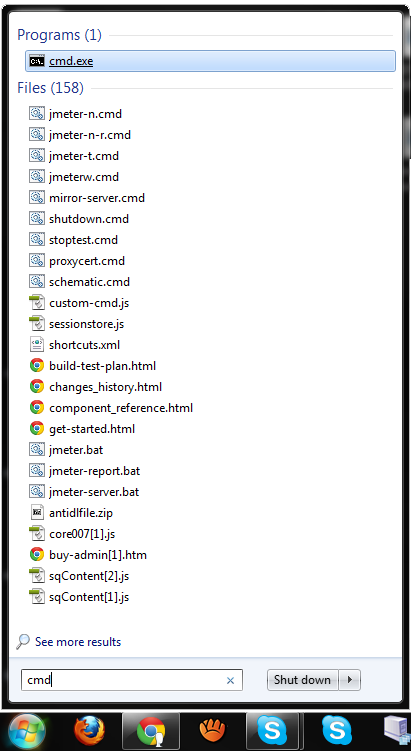2. Open your CMD and type in ipconfig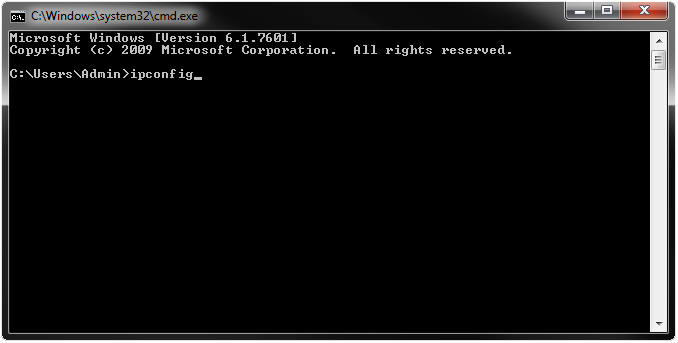3. You will get a lot of text.... Look at IPv4 Address. . . . . . . . . ***********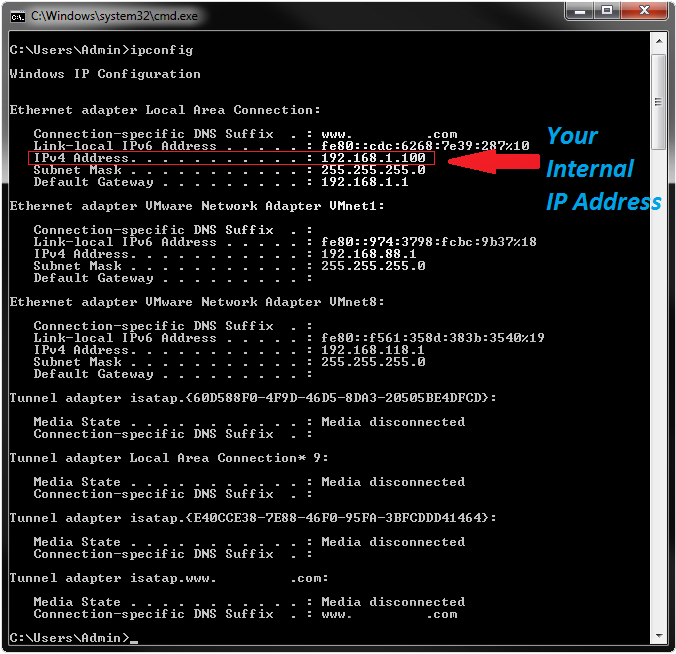4. As you can see on this image my Internal IP Address is: 192.168.1.100.

Written by: Maxym Maxymenko *(02.08.2012)*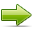IF YOU DID NOT UNDERSTAND SOMETHING, PLEASE LEAVE A COMMENT ON OUR FORUM